Two Interpretations of Division

Alignments to Content Standards: 3.OA.A.3

1. Maria cuts 12 feet of ribbon into 3 equal pieces so she can share it with her two sisters. How long is each piece?
2. Maria has 12 feet of ribbon and wants to wrap some gifts. Each gift needs 3 feet of ribbon. How many gifts can she wrap using the ribbon?

IM Commentary

Both of the questions are solved by the division problem $12 \div 3$ but what happens to the ribbon is different in each case. In the first case, the number of pieces of ribbon is fixed at 3 and the question is asking "how long is each piece?" (12 feet divided into 3 pieces gives 4 feet per piece.) In the second question, the size of each piece is fixed and the question is "how many pieces does one get?" (12 feet divided by 3 feet per gift gives 4 gifts.) The problem can be solved with a drawing of a tape diagram or number line. For problem 1, the line must be divided into 3 equal parts. The second problem can be solved by successive subtraction of 3 feet to see how many times it fits in 12.

In this case it is particularly helpful for the teacher to require students to justify their answers with a diagram. The way in which a student represents the problem can reveal whether or not he or she really understands the distinction between the two types of division problems shown here.

Solutions

Solution: Tape Diagram

1. This question asks, "how long is each piece?" so is a "How many in each group?" division problem: $$3\times ?= 12$$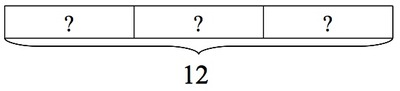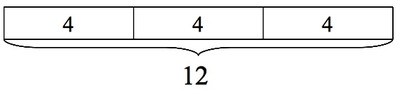$12 \div 3 = 4$, so each child gets a piece of ribbon that is 4 feet long.

2. This question asks, "how many pieces does one get?" so is a "How many groups?" division problem: $$? \times 3= 12$$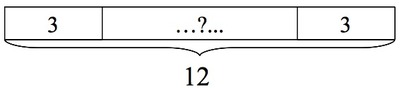$12 \div 3 = 4$, so Maria can wrap 4 gifts.

Solution: Number Line

1. This question asks, "how long is each piece?" so is a "How many in each group?" division problem: $$3\times ?= 12$$$12 \div 3 = 4$, so each child gets a piece of ribbon that is 4 feet long.

2. This question asks, "how many pieces does one get?" so is a "How many groups?" division problem: $$?\times 3= 12$$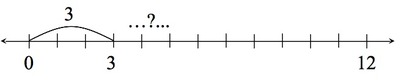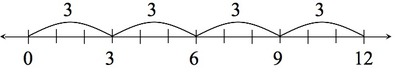$12 \div 3 = 4$, so Maria can wrap 4 gifts.